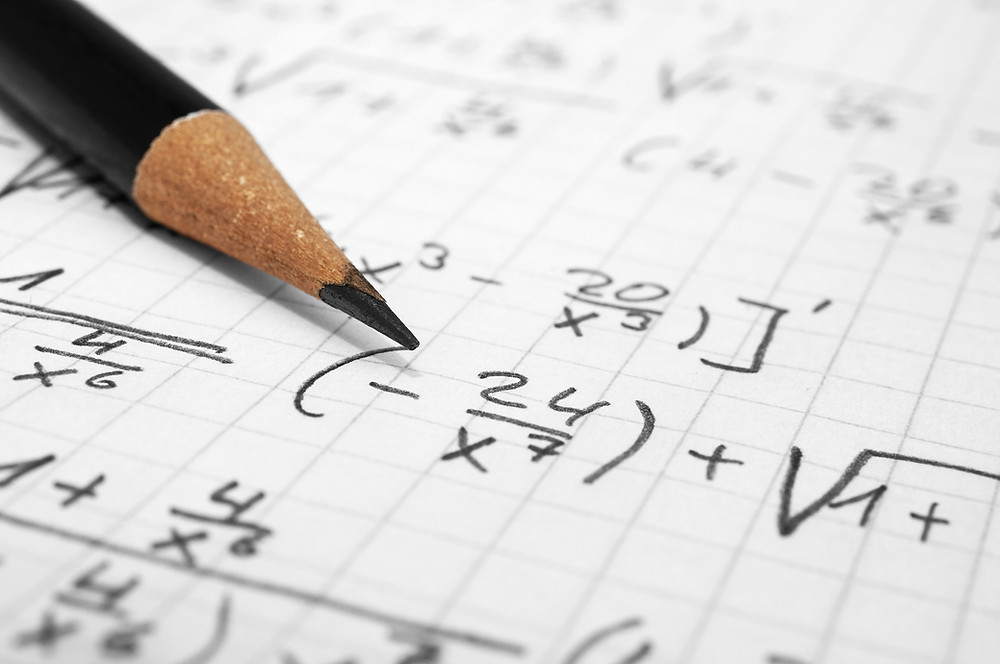Search
• rachit bakshi

# 5 Unsolved Mathematical Problems

There are numerous unsolved problems in mathematics. Some prominent unsolved problems include:P Versus NP Problem

A most famous problem in theoretical computer science.Its solution can deepen our understanding of algorithm complexity or maybe it will give us a mathematical tool for solving hard problems in an efficient way. An enormous amount of time has been spent on providing a solution but no one has ever been able to solve it.

Formally, P is the class of problems solved by a deterministic Turing machine in polynomial time. NP is the class of problems that are solved by a non-deterministic Turing machine in polynomial time, which means it can be solved by brute force algorithm in exponential time. We know that any P problem is also an NP one. In fact, the fast algorithm that solves the P problem can be its fast verification algorithm. The question is could we find an NP problem that doesn’t have a fast algorithm to solve it. If so then NP is different from P. If no then NP is identical to P.Most mathematicians and computer scientists expect that P≠NP.But the problem still remains unsolved.The Un-Knotting Problem

A knot is a closed simple (non-self-intersecting) curve embedded in the 3-dimensional space. Deciding if a particular is an unknot is a major driving force behind knot invariant since it was thought this approach would possibly give an efficient algorithm to recognize the knot from some presentations such as knot diagrams. Currently, there are several well-known unknot recognition algorithms(not using invariant), but they are either known to be inefficient or have no efficient implementation. Despite a great deal of effort, it is not known whether there exists an efficient algorithm for the un -knotting problem.

Navier-Stokes Existence and Smoothness

The Navier–Stokes Existence and Smoothness equations describe the motion of fluids. Although they were found in the 19th century, they still are not well understood. The problem is to make progress toward a mathematical theory that will give insight into this equation.

Goldbach’s Conjecture

Goldbach's conjecture is one of the oldest and best-known unsolved problems in number theory and all of mathematics. The conjecture is divided into two parts that are known as the weak and strong conjecture respectively. One part states that every even integer greater than 2 can be expressed as the sum of two primes. It has been verified for all even numbers to 400 trillion, but not yet proved.The other part of the definition of Goldbach’s Conjecture says that every odd whole number greater than 5 can be written as the sum of three primes.

The Large Cardinal Project

A large cardinal is a cardinal number that is larger than can be proven to exist in the ambient set theory. Large cardinals arrange themselves naturally into a more or less linear order of size and consistency strength and provide a convenient yardstick to measure the consistency strength of various other assertions that are Non provable.

## Recent Posts

See All

#### Education dept allows fee collection by Pvt schools; no clarity on the annual hike

Private schools in the national capital will not be allowed to increase fees during the corona virus lockdown without government consent and only the tuition fee can be charged until the time schools